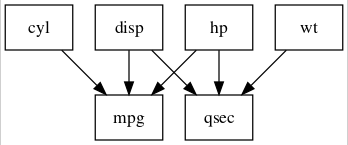Functions for embedding plots in Rmarkdown pdfs and for saving plots as pngs

embed_plot_pdf() saves your plot as a pdf image and then inserts that image into the pdf when you render Rmardown doc.

save_png() as the name suggests, saves your plot as a png image in the local directory (or wherever you tell it to).

model <- 'mpg ~ cyl + disp + hp
qsec ~ disp + hp + wt'

fit <- sem(model, data = mtcars)

pl <- lavaanPlot(model = fit)

# Example for pdf embed
embed_plot_pdf(pl, "plot2.pdf", width = 500)

# Example for saving to .png
save_png(pl, "plot.png", width = 500)

Now having saved the image, it can be embedded in the document with ![saved plot](plot.png)saved plot